NCERT Solutions: Introduction to Trigonometry (Exercise 8.2)

# NCERT Solutions: Introduction to Trigonometry (Exercise 8.2) Notes | Study Mathematics (Maths) Class 10 - Class 10

## Document Description: NCERT Solutions: Introduction to Trigonometry (Exercise 8.2) for Class 10 2022 is part of Mathematics (Maths) Class 10 preparation. The notes and questions for NCERT Solutions: Introduction to Trigonometry (Exercise 8.2) have been prepared according to the Class 10 exam syllabus. Information about NCERT Solutions: Introduction to Trigonometry (Exercise 8.2) covers topics like and NCERT Solutions: Introduction to Trigonometry (Exercise 8.2) Example, for Class 10 2022 Exam. Find important definitions, questions, notes, meanings, examples, exercises and tests below for NCERT Solutions: Introduction to Trigonometry (Exercise 8.2).

Introduction of NCERT Solutions: Introduction to Trigonometry (Exercise 8.2) in English is available as part of our Mathematics (Maths) Class 10 for Class 10 & NCERT Solutions: Introduction to Trigonometry (Exercise 8.2) in Hindi for Mathematics (Maths) Class 10 course. Download more important topics related with notes, lectures and mock test series for Class 10 Exam by signing up for free. Class 10: NCERT Solutions: Introduction to Trigonometry (Exercise 8.2) Notes | Study Mathematics (Maths) Class 10 - Class 10
 1 Crore+ students have signed up on EduRev. Have you?

1. Evaluate the following:
(i) sin 60° cos 30° + sin 30° cos 60°
(ii) 2 tan2 45° + cos2 30° – sin60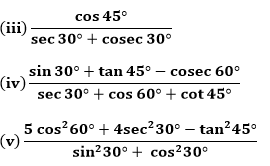Solution:
(i) The values of the given trigonometric ratios:
sin 30° = 1/2, cos 30° = √3/2, sin 60° = 3/2, cos 60°= 1/2
Now, substitute the values in the given problem
sin 60° cos 30° + sin 30° cos 60° = √3/2 ×√3/2 + (1/2) ×(1/2 ) = 3/4+1/4 = 4/4 = 1
(ii) The values of the trigonometric ratios:
sin 60° = √3/2, cos 30° = √3/2, tan 45° = 1
Substitute the values in the given problem
2 tan2 45° + cos2 30° – sin2 60 = 2(1)+ (√3/2)2-(√3/2)2 = 2 + 0 = 2
(iii) We know that:

cos 45° = 1/√2, sec 30° = 2/√3, cosec 30° = 2

Substitute the values, we get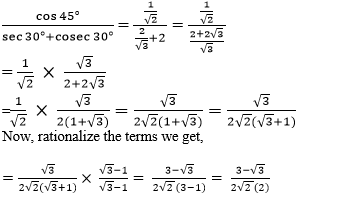Now, multiply both the numerator and denominator by √2, we get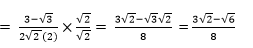Therefore, cos 45°/(sec 30°+cosec 30°) = (3√2 – √6)/8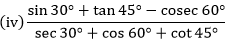We know that,
sin 30° = 1/2, tan 45° = 1, cosec 60° = 2/√3, sec 30° = 2/√3, cos 60° = 1/2, cot 45° = 1
Substitute the values in the given problem, we get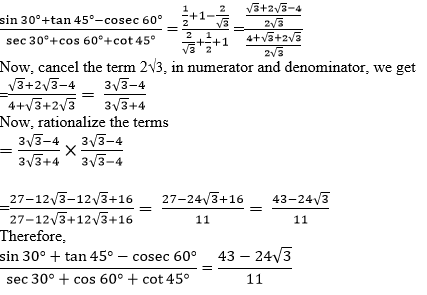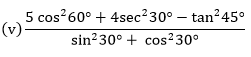We know that,
cos 60° = 1/2, sec 30° = 2/√3, tan 45° = 1, sin 30° = 1/2, cos 30° = √3/2
Now, substitute the values in the given problem, we get
(5cos260° + 4sec230° – tan245°)/(sin30° + cos30°)
= 5(1/2)+ 4(2/√3)- 12/(1/2)+ (√3/2)2 = (5/4 + 16/3 - 1)/(1/4 + 3/4) = {(15 + 64 - 12)/12}/(4/4) = 67/12

2. Choose the correct option and justify your choice:
(i)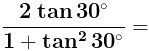(a) sin 60°
(b) cos 60°
(c) tan 60°
(d) sin 30°
Ans. (A) is correct
Justification: Substitute tan 30° = 1/√3 in the given equation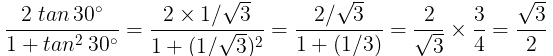∵ √3/2 = sin 60°
The obtained solution is equivalent to the trigonometric ratio sin 60°

Question for NCERT Solutions: Introduction to Trigonometry (Exercise 8.2)
Try yourself:(ii)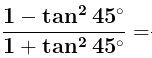(iii)  sin 2A = 2 sin A is true when A =
(a) 0°

(b) 30°
(c) 45°
(d) 60°
Ans. (A) is correct.
Justification: To find the value of A, substitute the degree given in the options one by one
sin 2A = 2 sin A is true when A = 0°
As, sin 2A = sin 0° = 0 and 2 sin A = 2 sin 0° = 2 × 0 = 0
or,
Apply the sin 2A formula, to find the degree value
sin 2A = 2sin A cos A
⇒ 2sin A cos A = 2 sin A
⇒ 2cos A = 2 ⇒ cos A = 1
Now, we have to check, to get the solution as 1, which degree value has to be applied.
When 0 degree is applied to cos value, i.e., cos 0 = 1
Therefore, ⇒ A = 0°

Question for NCERT Solutions: Introduction to Trigonometry (Exercise 8.2)
Try yourself:(iv)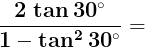3. If tan (A + B) = √3 and tan (A – B) = 1/√3 ,0° < A + B ≤ 90°; A > B, find A and B.
Solution:
tan (A + B) = √3
Since √3 = tan 60°
Now substitute the degree value
⇒ tan (A + B) = tan 60°
(A + B) = 60° … (i)
The above equation is assumed as equation (i)
tan (A – B) = 1/√3
Since 1/√3 = tan 30°
Now substitute the degree value
⇒ tan (A – B) = tan 30°
(A – B) = 30° … equation (ii)
Now add the equation (i) and (ii), we get
A + B + A – B = 60° + 30°
Cancel the terms B
2A = 90°
A= 45°
Now, substitute the value of A in equation (i) to find the value of B
45° + B = 60°
B = 60° – 45°
B = 15°
Therefore A = 45° and B = 15°

4. State whether the following are true or false. Justify your answer.
(i) sin (A + B) = sin A + sin B.
Ans. False
Justification: Let us take A = 30° and B = 60°, then
Substitute the values in the sin (A + B) formula, we get
sin (A + B) = sin (30° + 60°) = sin 90° = 1 and,
sin A + sin B = sin 30° + sin 60°
= 1/2 + √3/2 = 1+√3/2
Since the values obtained are not equal, the solution is false.

(ii) The value of sin θ increases as θ increases.
Ans. True
Justification: According to the values obtained as per the unit circle, the values of sin are: sin 0° = 0, sin 30° = 1/2, sin 45° = 1/√2, sin 60° = √3/2, sin 90° = 1
Thus the value of sin θ increases as θ increases. Hence, the statement is true
(iii) The value of cos θ increases as θ increases.
Ans. False
Justification: According to the values obtained as per the unit circle, the values of cos are: cos 0° = 1, cos 30° = √3/2, cos 45° = 1/√2, cos 60° = 1/2, cos 90° = 0
Thus, the value of cos θ decreases as θ increases. So, the statement given above is false.
(iv) sin θ = cos θ for all values of θ.
Ans. False
Justification: sin θ = cos θ, when a right triangle has 2 angles of (π/4). Therefore, the above statement is false.
(v) cot A is not defined for A = 0°.
Ans. True
Justification: Since cot function is the reciprocal of the tan function, it is also written as:
cot A = cos A/sin A
Now substitute A = 0°
cot 0° = cos 0°/sin 0° = 1/0 = undefined.
Hence, it is true

The document NCERT Solutions: Introduction to Trigonometry (Exercise 8.2) Notes | Study Mathematics (Maths) Class 10 - Class 10 is a part of the Class 10 Course Mathematics (Maths) Class 10.
All you need of Class 10 at this link: Class 10

## Mathematics (Maths) Class 10

53 videos|369 docs|138 tests
 Use Code STAYHOME200 and get INR 200 additional OFF

## Mathematics (Maths) Class 10

53 videos|369 docs|138 tests

Track your progress, build streaks, highlight & save important lessons and more!

,

,

,

,

,

,

,

,

,

,

,

,

,

,

,

,

,

,

,

,

,

;